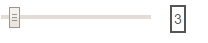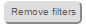Old Versions ( v1.0|v1.1|v1.2 )

## TMDL Report Selection Tool v 1.3

please cite as: Kumar, S. (2016). TMDL Report Selection Tool (TRS). Retrieved curdate, from https://occviz.com/tmdl

States
EPA Regions

Downloading and processing 35MB dataset. May take time.This app is being developed by Saurav Kumar ( email: kumar.saurav+tmdl@gmail.com). Drop me a note if you find this useful or to suggest changes.

## How to use this app

This app assists with filtering the table of TMDL reports based on your interests. There are two steps to using this app:

1. Select the "critical frequency" using the slider on the top right of this page.This slider controls how reports are classified as having used one or more models. For example, if the selected value for the slider is "30", then any model name (and its derivatives or alternates; see notes on regex later) has to occur 30 or more times in the text of that report, for the report to be marked as using that model. Notice as you move the slider to a higher number, fewer reports are available. This slider has no impact on impairment. Impairment labels were included in the metadata about the report from EPA and assumed to be correctly classified.
2. Click on the groups in the chord graph to filter reports and investigate relationships. Clickbutton anytime to clear all filters.

## Data generation

1. All the TMDL report data were obtained from USEPA ATTAINS database  LINK to ATTAINS.
2. The textual contents of the reports obtained (pdfs) were searched (after OCR, if needed) for occurrences of models covered by ASCE EWRI TMDL Analysis and Modeling Task Committee and other terms associated with TMDL using following "regex". Use  https://regex101.com/#python to test these regex; note the text after the colon is the regex.
`   "mass balance":"(?i)mass(.{0,1}\s{0,1})(?i)balance(.{0,1}\s{0,1})(?i)model",`
`    "simple method":"(?i)simple(.{0,1}\s{0,1})((?i)method|(?i)model)",`
`    "bathub":"(\s|\(|\))(?i)bathub",`
`    "sstemp":"(\s|\(|\))(?i)sstemp",`
`    "load duration curve":"(((?i)load|(?i)flow)(.{0,1}\s{0,1})(?i)duration(.{0,1}\s{0,1})(?i)curve|\sldc\s)",`
`    "usle":"(\s|\(|\))(USLE|RUSLE)",`
`    "agnps":"(\s|\(|\)|(?i)ann(-{0,1}|\s{0,1}))(?i)agnps",`
`    "answers":"(\s|\(|\))ANSWERS",`
`    "dwsm":"(\s|\(|\))(?i)dwsm",`
`    "gssha":"(\s|\(|\))(?i)gssha",`
`    "gwlf":"(\s|\(|\))((?i)gwlf|(?i)basinsim)",`
`    "hec-hms":"(\s|\(|\))(?i)hec(\s|-)(?i)hms",`
`    "hspf":"(\s|\(|\))((?i)hspf|(?i)lspc)",`
`    "basins":"(\s|\(|\))BASINS",`
`    "kineros":"(\s|\(|\))(?i)kineros",`
`    "mike she":"(\s|\(|\)|)(?i)mike(.{0,1}\s{0,1})(?i)she",`
`    "stepl":"(\s|\(|\))(?i)stepl",`
`    "spreadsheet":"(\s|\(|\))(?i)spreadsheet(\s|\(|\)|-)",`
`    "swat":"(\s|\(|\))(?i)swat",`
`    "swmm":"(\s|\(|\)|-)(?i)swmm",`
`    "warmf":"(\s|\(|\))(?i)warmf",`
`    "curve number":"(\s|\(|\))(?i)curve(.{0,1}\s{0,1})(?i)number(\s|\(|\)|-)",`
`    "cche":"(\s|\(|\))(?i)cche",`
`    "cequalriv":"(?i)ce(.{0,1}\s{0,1})(?i)qual(.{0,1}\s{0,1})(?i)riv",`
`    "cequalw2":"(?i)ce(.{0,1}\s{0,1})(?i)qual(.{0,1}\s{0,1})(?i)w2",`
`    "concepts":"(\s|\(|\))CONCEPTS",`
`    "efdc":"(\s|\(|\))(?i)efdc",`
`    "edp riv1":"(\s|\(|\))(?i)edp(.{0,1}\s{0,1})(?i)riv",  # riv has number`
`    "hec ras":"(\s|\(|\))(?i)hec(.{0,1}\s{0,1})(?i)ras",`
`    "mike 11":"(\s|\(|\))(?i)mike(.{0,1}\s{0,1})(?i)11",`
`    "minteq":"(\s|\(|\))((?i)mineql|(?i)minteq)", # no end space `
`    "oteq": "(\s|\(|\))(?i)oteq",`
`    "qual":"(\s|\(|\))(?i)qual(.{0,1}\s{0,1})(?i)2e",`
`    "qual":"(\s|\(|\))(?i)qual(.{0,1}\s{0,1})(?i)2k",`
`    "wasp":"(\s|\(|\))(?i)wasp",`
`    "sustain":"(\s|\(|\))SUSTAIN",`
`    "tidal prism":"(\s|\(|\))(?i)tidal(.{0,1}\s{0,1})prism",`
`    "mos" : "(\s|\(|\))(MOS|mos|margin of safety)",`
`    "explicit": "(\s|\(|\))(?i)explicit(.{0,1}\s{0,1})mos",`
`    "implicit": "(\s|\(|\))(?i)implicit(.{0,1}\s{0,1})mos",`
`    "remote sensing": "(\s|\(|\))(?i)remote(.{0,1}\s{0,1})sensing",`
`    "GIS": "(\s|\(|\))(GIS)",`